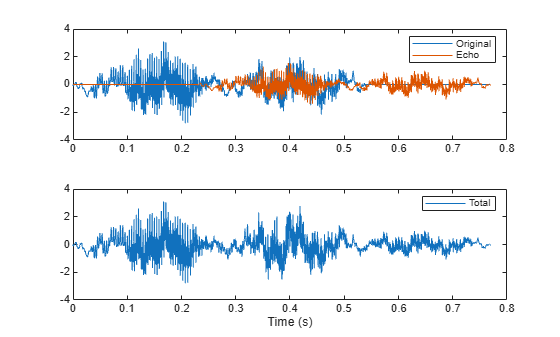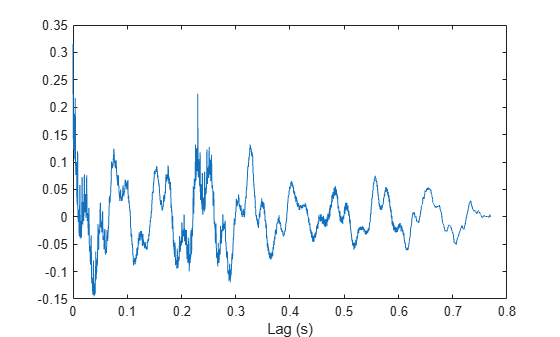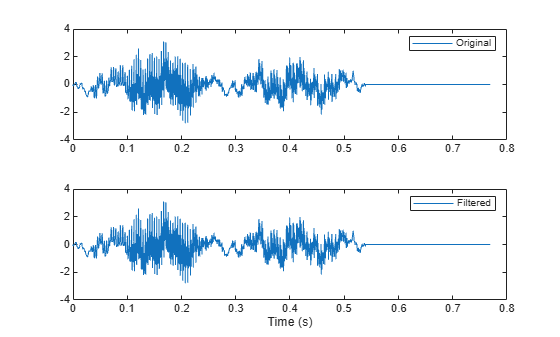# Echo Cancelation

A speech recording includes an echo caused by reflection off a wall. Use autocorrelation to filter it out.

In the recording, a person says the word MATLAB®. Load the data and the sample rate, ${F}_{s}=7418\phantom{\rule{0.2777777777777778em}{0ex}}Hz$.

```load mtlb % To hear, type soundsc(mtlb,Fs)```

Model the echo by adding to the recording a copy of the signal delayed by $\Delta$ samples and attenuated by a known factor $\alpha$: $y\left(n\right)=x\left(n\right)+\alpha x\left(n-\Delta \right)$. Specify a time lag of 0.23 s and an attenuation factor of 0.5.

```timelag = 0.23; delta = round(Fs*timelag); alpha = 0.5; orig = [mtlb;zeros(delta,1)]; echo = [zeros(delta,1);mtlb]*alpha; mtEcho = orig + echo;```

Plot the original, the echo, and the resulting signal.

```t = (0:length(mtEcho)-1)/Fs; subplot(2,1,1) plot(t,[orig echo]) legend('Original','Echo') subplot(2,1,2) plot(t,mtEcho) legend('Total') xlabel('Time (s)')````% To hear, type soundsc(mtEcho,Fs)`

Compute an unbiased estimate of the signal autocorrelation. Select and plot the section that corresponds to lags greater than zero.

```[Rmm,lags] = xcorr(mtEcho,'unbiased'); Rmm = Rmm(lags>0); lags = lags(lags>0); figure plot(lags/Fs,Rmm) xlabel('Lag (s)')```The autocorrelation has a sharp peak at the lag at which the echo arrives. Cancel the echo by filtering the signal through an IIR system whose output, $w$, obeys $w\left(n\right)+\alpha w\left(n-\Delta \right)=y\left(n\right)$.

```[~,dl] = findpeaks(Rmm,lags,'MinPeakHeight',0.22); mtNew = filter(1,[1 zeros(1,dl-1) alpha],mtEcho);```

Plot the filtered signal and compare to the original.

```subplot(2,1,1) plot(t,orig) legend('Original') subplot(2,1,2) plot(t,mtNew) legend('Filtered') xlabel('Time (s)')````% To hear, type soundsc(mtNew,Fs)`